## Introduction

In the past years much interest has been devoted to the study of light beams with a spatially inhomogeneous phase profile like, for instance, eigenstates of the orbital angular momentum (OAM). In general, light can carry both spin and OAM: the first is associated with circular polarizations; the second corresponds to helical wavefronts, as described mathematically by an azimuthal phase factor of the form exp(ilφ), where φ is the azimuthal angle in a cylindrical coordinate system around the wave propagation axis z and the topological charge l represents the integer number of crossed helical wavefronts when circling around the axis once. Hence, a circularly polarized photon state with helical wavefront carries a spin of σħ(with σ = ±1) and an OAM of lħ1. Typical examples of OAM eigenstates are Laguerre-Gauss or Bessel-Gauss spatial transverse modes2. Generation of helical waves requires optical devices that introduce a local phase shift on the beam cross section. Here we consider a particular liquid-crystal-based device, called q-plate (QP)3, which has so far been successfully exploited for generation and detection of OAM eigenstates in a variety of applications4, ranging from metrology5 to fundamental quantum mechanics6. Moreover, OAM provides a wide alphabet for qudit encoding for quantum information and computation purposes7.

OAM can also be exploited to describe the properties of a class of light beams characterized by a polarization that varies along the azimuthal coordinate φ: the so-called vector vortex (VV) beams, also known as spirally-polarized beams. Generation and manipulation of such inhomogeously polarized light beams has been carried out with several techniques, both in the classical8,9,10 and single photon regime11,12. An important subfamily of VV beams are those having cylindrical-symmetric polarization patterns, including in particular radial and azimuthal polarized beams13. These two last states can be expressed for instance as suitable linear combinations of two helical waves with opposite topological charge l = +1 and l = −1 and opposite (uniform) circular polarizations. More in general, a linear combination of two OAM eigenstates with eigenvalue ±l can give rise to an arbitrary VV beam8. Symmetric pairs of VV beams in turn define, by linear combination, two-dimensional spaces of non-uniform polarization states formally analogous to the standard Poincaré sphere, which have been named in the literature as “hybrid Poincaré spheres” (HPS)14 or “higher-order Poincaré spheres”15. Such inhomogenous polarization states have particular properties that can be exploited to produce strong longitudinal field components16 or to obtain a tight focusing17. This leads to applications in particle acceleration18, spectroscopy19, microscopy20, optical trapping21 and others. Moreover, in a single particle regime, VV beams can be viewed as entangled states between spin and orbital angular momentum degrees of freedom22,23 and can be expressed via a geometric representation of entangled qubits24,25,26,27,28. It has also been recently shown that particular VV states can be exploited for enhanced sensitivity in angular measurements5 and rotationally-invariant quantum communication29,30. This last result follows from a proposal by Aolita and Walborn31 and a proof of principle in classical regime that has been carried out by Souza and co-workers by simulating a physical rotation of reference frame via birefringent waveplates32.

In this Letter we introduce and experimentally test a technique, exploiting a particular kind of q-plates with controllable phase-retarding action, that allows a precise and arbitrary manipulation of VV states while remaining within a given HPS space. In other words, we demonstrate arbitrary transformations within these spheres, analogous to the quantum transformations that can be applied on two-state quantum systems, as represented on a Bloch sphere. A pure polarization-to-VV beam conversion process and its reverse one are used for generation and analysis of these states, in order to study the rotation action by means of standard polarization tomography.

## Results

### Q-plates general description

Q-plates are liquid-crystal devices that act as interfaces between homogeneously polarized and VV beams via a spin-orbit coupling of the angular momentum of light3,4.

A QP is a birefringent plate with a uniform birefringent phase retardation δ and characterized by an optic axis transverse distribution having a singular pattern with topological charge q and a given initial orientation α0. The optic axis pattern is described by the following expression: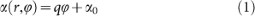where the QP is supposed lying on the xy plane, described in polar coordinates (r, φ) and α denotes the angle formed by the optic axis with the x axis at each point in the plate. Examples of q-plate geometries are shown in Fig. 1. An initially Gaussian circular polarized beam passing through the q-plate is partially or totally converted, depending on the value of δ, into a beam carrying an OAM of ±2qħ per photon, with a flipped polarization handedness. More precisely, the QP action on hybrid spin-orbital angular momentum eigenstates can be expressed as follows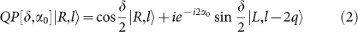and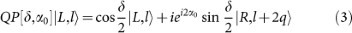where |π, l〉 represents a photon state of polarization π and carrying an OAM of lħ. Equation (2) and (3) show that, by acting on the retardation δ, it is possible to vary the coefficients of the modified and unmodified OAM components. Retardation manipulations can be accomplished in several ways, such as by applying pressure, varying the material temperature or, most conveniently, by applying an external electric field33. Most of the applications for such optical devices have so far exploited optimal tuning (δ = π) to achieve a complete encoding-decoding process between homogeneous polarization photon states and vector beams5,29,34. The encoding-decoding system consists in two q-plates with the same topological charge, where the first one converts a given homogeneous polarization state in the corresponding VV and the second one transform the VV back to the homogeneous polarization state. In this way, it is possible to generate and measure VV states with standard optical polarization techniques. The set of the two encoding/decoding q-plates can be considered as a unique device, represented in the {|R, 0〉, |L, 0〉} basis as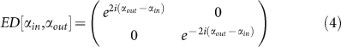where αin and αout denote the α0 for the geometry of the first and second q-plate, respectively. Thus, the only visible effect on a linearly polarized beam passing through the device (4) is a polarization rotation of 2(αout−αin), rotation which can be easily avoided by rotating one of the q-plates around the z axis until αout = αin. The only rotationally invariant geometry is the q = 1 case, where the α0 value, corresponding to the φ = 0 optic axis orientation, has to be set in the production process. Hence, for this particular geometry, the condition αout = αin is automatically satisfied once the two q-plates are manufactured in the same way.

### Manipulating vector beams with q-plates

We now show how a q-plate can be exploited to directly manipulate a vector beam state while remaining in a given HPS by acting on the OAM degree of freedom and polarization simultaneously. Suppose that a photon is prepared in a VV state, which can be expressed in the HPS representation15 (see Fig. 1) as follows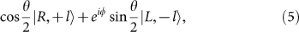where θ and ϕ are the polar coordinates of the state in the HPS. Consider then a QP with optical retardation δ and topological charge q = l acting on the photon state. Through (2) and (3), the transformation applied by the device can be written in the chosen logical basis as: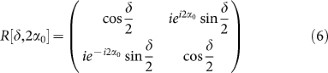Such unitary transformation represents a logical clockwise state rotation of angle δ on the HPS sphere, with rotation axis lying on the xy plane (i.e., rotation axis polar angle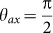), with the angle α0 that fixes the azimuthal angle of the axis, according to ϕax = −2α0. This can be shown by considering for instance an incoming |R, +l〉 state that by (6) is transformed into: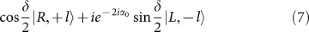The state trajectory thus follows the meridian corresponding to ϕ = −2α0 + π/2, with θ varying exactly as δ. Moreover, the rotation action described by equation (6) can be exploited to achieve an arbitrary state transformation. Indeed, to reach the state of coordinates {θ1, ϕ1} starting from an initial state {θ0, ϕ0}, it is enough to choose the rotation axis (and therefore to set the α0 value) so as to have the two states belonging to the same rotation plane. In this way the rotation trajectory connects the two states and the desired transformation can be accomplished, provided the proper δ value is chosen. (Another possible method consists in combining two QP with proper initial axis orientations, their corresponding rotation matrices chosen to be R[−θ0, π/2 − ϕ0] and R1, π/2 − ϕ1]. The first transformation converts the initial {θ1, ϕ1} state in the |R, +l〉 one and then the second coincides with (7), δ and α0 values chosen in order to reach the desired final coordinates). As already pointed out, the two parameters α0 and δ can be easily controlled by rotating the q-plate and tuning it respectively, hence an arbitrary transformation is easy to obtain. The only q-plate geometry that does not allow the α0 manipulation via rotation is the q = 1 one, since it is rotationally invariant. In this case α0 can be controlled in the manufacturing process as explained in the next section. However, even without a dynamic control of α0, it is still always possible to perform an arbitrary transformation on the HPS by using three q-plates (or two, if their order can be exchanged), as explained in the Supplementary Information.

### Experiment

Here we describe the first experimental implementation of the rotating device (6) corresponding to an electrically tuned QP with topological charge q = 1 and α0 = π/4. This device allows one to perform a logical rotation on the HPS of cylindrically-symmetric beams around the direction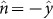. The experimental setup is shown in Fig. 2. A laser beam produced by a Ti-Sapphire pulsed laser with wavelength λ = 795nm is spectrally purified by interference filters with bandwidth Δλ = 3 nm and projected onto the spatial mode TEM00 by a single mode fiber. The beam is then projected onto the linear polarization state |H〉 by a polarizing beam splitter (PBS) and then transformed into an arbitrary polarization state via birefringent waveplates. A pure polarization-to-VV beam conversion process and its reverse one are accomplished by means of two completely tuned q = 1/2 q-plates, the first one acting as encoder and the second one as decoder. In the first part of the experiment we characterized the electrical response of the q = 1 q-plate in order to retrieve the retardation δ corresponding to a given applied voltage33. By removing the two q = 1/2 q-plates from the setting, we measured the intensity of converted (Ic) and unconverted (Iu) components for a circular polarized input beam, as functions of the applied voltage. From (2,3) we know that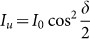(where I0 stands for the total output intensity), hence we recovered the δ values corresponding to each applied voltage (see Fig. 2b).

We then verified the logical rotation action of the intermediate q-plate for various input states. Our experimental configuration allowed us to visualize the rotation, acting on the VV beam, as a pure polarization one, implying that state preparation as well as projective measurements could be performed by means of ordinary polarization optics. Thus we observed the rotation through polarization tomography of the output state corresponding to different δ values, ranging from the case of detuned q-plate (δ = 0) to complete tuning (δ = π). By considering the additional phase retardation induced by the q = 1/2 codifying system, it is easy to show that the global three-q-plate device action is represented in the circular polarization basis, apart from global phase factors, by the following matrix: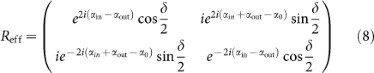The linear polarization rotation effect is clearly visible in the δ = 0 case, since (8) corresponds exactly to (4). To cancel such effect, we chose αin − αout = 0. This can be done by detuning the q = 1 q-plate and rotating the first q = 1/2 with respect to the others (thus varying αin) until an incoming horizontally polarized beam remains unaffected after passing through the system. We point out that by following this method, one ends up with two q = 1/2 sharing the same initial optic axis orientation. Such initial orientation is in general different from that of the q = 1 one (α0 ≠ αin), this causing a discrepacy between the expected rotation axis, associated with α0 and the effective rotation visualized in polarization by means of the codifying system. Indeed, by posing αin = αout = α1, the (8) coincides with (6) and an effective rotation axis angle ϕax = 2α0 − 4α1. Fig. 3 reports our experimental results, showing the rotation effect on the Poincaré sphere of polarizations, mapping the equivalent rotation in the HPS.

It can be seen that the rotation planes for each input state are almost parallel, confirming that all the trajectories share the same rotation axis, identified by the red arrow. Since we exploited an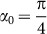q-plate, such axis was expected to be exactly the diagonal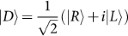state. The effective rotation axis is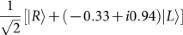and the discrepacy between expected and obtained result can be ascribed to an α1 = −0.084. In order to check the control achievable on the VV state manipulation, we compared the experimental results to the expected trajectories calculated from (8) in correspondence of the same δ values and with the α1 found. The resulting fidelities between experimental and theoretical states are shown in Fig. 2, corresponding to a mean value of F = 0.99485 ± 0.00006. We point out that in general, the visualized rotation axis can be modified in order to coincide with the real one by accordingly rotating both q = 1/2 q-plates with respect to the q = 1 one, thus varying α1. The desired result (in our case a rotation axis parallel to |D〉) is achieved when α1 = 0.

As a last step, we wanted to confirm that the state manipulation acts on VV beams and not on polarization. Our experimental test was performed on states (5) with l = 1, which are rotationally invariant, i.e. independent of the relative angle formed by generation and detection setups around the propagation axis. Therefore we mounted the state tomography setup on a rotational stage that can be rotated along the light propagation axis and we repeated measurements for several misalignement angles between the state preparation stage and the state tomography one29. Fig. 3 shows that the rotation axis does not depend on the relative rotation between generation and detection stages, thus the rotation action is necessarily acting on the VV beam's state, since the reference frame independence is preserved.

## Discussion

We introduced a technique that allows the arbitrary manipulation of the inhomogeneous state of polarization of vector vortex beams by means of q-plates with controllable parameters such as tuning and initial optic axis orientation. Our experimental implementation considered a single rotating device acting on VV beams arising form l = ±1 OAM eigenstates, corresponding to cylindrically symmetric beams and we showed that manipulation is indeed achievable with high fidelity independently of the input state considered. Interesting developments could include testing the arbitrary rotation induced by a couple of q-plates with orthogonal rotation axes, as well as exploiting the same manipulation for states of polarization associated with a combination of higher OAM values. Moreover, we point out that alignement-free quantum communication29 is related to qubit encoding anologous to (5) with l = 1. Therefore our work paves the way to manipulation of rotationally-invariant photonic qubits without requiring an encoding-decoding system.

## Methods

### Q-plate fabrication methods

The q-plates were manufactured by aligning a nematic liquid crystal (LC) material with the orientation angle given in Eq. (1). The desired planar alignment of the LC was induced using a photoalignment technique35. We used 0.1% wt. solution of Brilliant Yellow (BY) dye in dimethylformamide (DMF) as the aligning surfactant and Indium-Tin-Oxide (ITO) coated glass substrates so to have the possibility of applying an external electrical field to the LC film. For preparing the alignment pattern, a polarized UV laser beam was expanded by a set of lenses, sent through a half-wave plate to set its polarization orientation and focused with a cylindrical lens, so as to illuminate only a thin azimuthal sector of the LC cell. Both the waveplate and the sample were attached to rotating mounts controlled by a computer, so as to impress sequentially the desired alignment to each azimuthal sector of the LC cell.